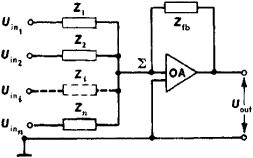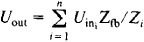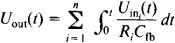# Computing Amplifier

## Computing Amplifier

in analogue computers, a composite device consisting of a DC amplifier and external components that form a feedback circuit. The computing amplifier is designed to perform certain mathematical operations on analogue quantities—for example, addition, integration, differentiation, and multiplication by constants. For this reason, the amplifier proper, without the feedback circuit, is called an operational amplifier. Various types of computing amplifiers exist, such as pneumatic, hydraulic, and magnetic amplifiers. The most common type is the electronic computing amplifier, in which voltages or currents are used as signals.Figure 1. Block diagram of a computing amplifier: (Uin1), (UIN2), (UIN3) and (Uinn) voltages (signals) at inputs of computing amplifier; (Z1), (Z2), (Zt), and (Zn) input resistors; (Σ) summing junction; (Zfb) feedback resistor; (Uout) output voltage (signal); (OA) operational amplifier

When one or more input voltages appear at the inputs of the computing amplifier (Figure 1), the currents I1, …, In flow through the input resistors and are summed at the point Σ, the summing junction, at the input of the operational amplifier. Because the gain of the operational amplifier is very high, the voltage at Σ is practically equal to zero. For this reason, I1 + … + In = Ifb. Since, however,

I1 = Uin1/Z1, …, Iin n/Zn

and Ifb = Uout/Zfb, it follows thatThe ratio Zfb/Zi determines the mathematical operation performed on the input i. If Zfb/Z1 = 1, then the computing amplifier performs an algebraic summation of the input voltages. If Zfb/Zi = ki, where Zi and are resistances, then the summation is performed with the simultaneous multiplication of the summands by the constants ki. If impedors are included in the feedback circuit, there occurs a more complicated transformation of the input signals over time. Suppose, for example, the Zi are resistors (equal to Ri•), and the feedback circuit is formed by a capacitor Cfb. Then,that is, the sum of the input voltages is integrated with respect to time. When nonlinear resistors are used in the feedback circuits, computing amplifiers permit the performance of such nonlinear operations as raising to a power, the finding of trigonometric functions, and the multiplication of variables.

There are three principal causes of error in the performance of operations by a computing amplifier: inaccuracy of the ratings of the feedback-circuit components, instability of the components, and imperfection of the operational amplifier. The higher the gain ka and the input resistance of the operational amplifier and the lower the output resistance of the operational amplifier, the smaller the error. A considerable influence on the error is had by the parasitic input current Ip generated by the operational amplifier, the zero shift Ep, and the instability in these factors—drift with time and changing temperature (seeDRIFT, ZERO-LEVEL); noise also contributes to the error. The dynamic error of a computing amplifier will be less where the bandwidth is wider, the cutoff frequency fco (at which ka~ 1) is higher, and the rise rate of Uout is greater.Figure 2. Block diagram of an operational amplifier: (In) input of the operational amplifier, (C1) and (C2) coupling capacitors, (A1) low-frequency and DC amplifier, (A2) high-frequency amplifier with ka~ 1, (A3) medium-frequency amplifier, (A4) wide-band output amplifier, (Out) output of the operational amplifier

High-quality operational amplifiers are usually built with several parallel amplification channels (Figure 2). Such operational amplifiers provide ka= 108–109, Ip = 10–12-10-10 amperes, Eρ= 1–50 microvolts, and fco=1–100 megahertz. Operational amplifiers with one amplification channel have ka= 104-106, IP= 10-11-10-6 amperes, and fco = 1–20 megahertz.

### REFERENCES

Polonnikov, D. E. Reshaiushchie usiliteli. Moscow, 1973.
Proektirovanie i primenenie operatsionnykh usilitelei. Moscow, 1974. (Translated from English.)

D. E. POLONNIKOV

Site: Follow: Share:
Open / Close